# Trigonometry : Trigonometry

## Example Questions

← Previous 1 3 4 5 6 7 8 9 54 55

### Example Question #2 : Trigonometric Identities

Which of the following trigonometric identities is INCORRECT?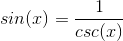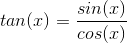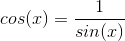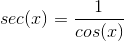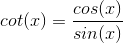Explanation:

Cosine and sine are not reciprocal functions.and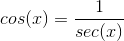### Example Question #1 : Trigonometric Identities

Using the trigonometric identities prove whether the following is valid: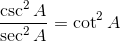Uncertain

Only in the range of: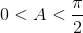True

False

Only in the range of: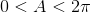True

Explanation:

We begin with the left hand side of the equation and utilize basic trigonometric identities, beginning with converting the inverse functions to their corresponding base functions: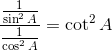Next we rewrite the fractional division in order to simplify the equation: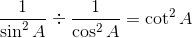In fractional division we multiply by the reciprocal as follows: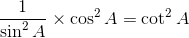If we reduce the fraction using basic identities we see that the equivalence is proven: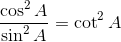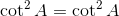### Example Question #1 : Trigonometric Identities

Using trigonometric identities determine whether the following is valid: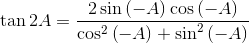True

Uncertain

Only valid in the range of:False

Only valid in the range of:False

Explanation:

We can choose either side to work with to attempt to obtain the equivalency. Here we will work with the right side as it is the more complex. First, we want to eliminate the negative angles using the appropriate relations. Sine is odd and therefore, the negative sign comes out front. Cosine is even which is interpreted by dropping the negative out of the equation: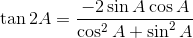The squaring of the sine in the denominator makes the sine term positive, i.e.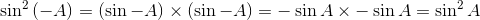The numerator is the double angle formula for sine: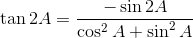The denominator is recognized to be the pythagorean theorem as it applies to trigonometry: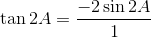The final reduced equation is: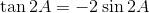Thus proving that the equivalence is false.

### Example Question #1 : Basic And Definitional Identities

Which of the following describes the ratio of sine?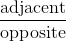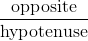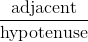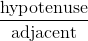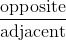Explanation:

Sine is by definition of sides in a right triangle is opposite side over hypotenuse.

To remember this, use SOH CAH TOA.

SOH: Sine=Opposite/Hypotenuse

### Example Question #1 : Trigonometric Identities

Which of the following is the best answer for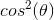?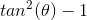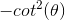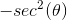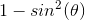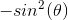Explanation:

Write the Pythagorean identity.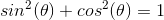Substract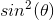from both sides.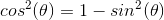### Example Question #1 : Trigonometry

If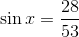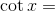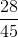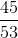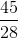Explanation:

The sine of an angle in a right triangle (that is not the right angle) can be found by dividing the length of the side opposite the angle by the length of the hypotenuse of the triangle.

From this, the length of the side opposite the angleis proportional to 28, and the length of the hypotenuse is proportional to 53.

Without loss of generality, we'll assume that the sides are actually of length 28 and 53, respectively.

We'll use the Pythagorean theorem to determine the length of the adjacent side, which we'll refer to as.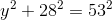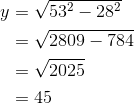The cotangent of an angle in a right triangle (that is not the right angle) is can be found by dividing the length of the adjacent side by the length of the opposite side.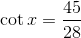### Example Question #8 : Trigonometric Identities

State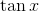in terms of sine and cosine.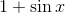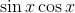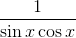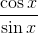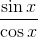Explanation:

The definition of tangent is sine divided by cosine.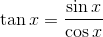### Example Question #9 : Trigonometric Identities

Simplify.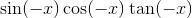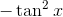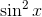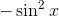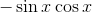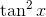Explanation:

Using these basic identities: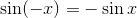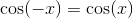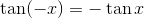we find the original expression to be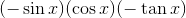which simplifies to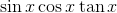.

Further simplifying: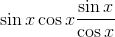The cosines cancel, giving us### Example Question #1 : Trigonometric Identities

Which of the following identities is incorrect?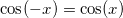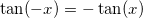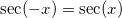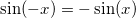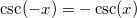Explanation:

The true identity is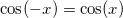because cosine is an even function.

### Example Question #1 : Pythagorean Identities

Simplify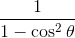.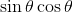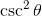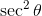Explanation:

To simplify, recognize that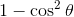is a reworking on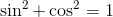, meaning that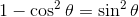.

Plug that into our given equation: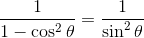Remember that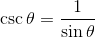, so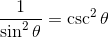.

← Previous 1 3 4 5 6 7 8 9 54 55

### All Trigonometry Resources